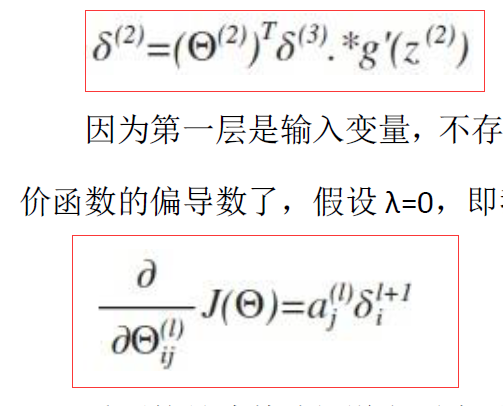delte2=theta2_non'*delte3;%第二层误差,行

delte2=delte2.*a2(2:end,:).*(1-a2(2:end,:));%完全版误差公式`````` clc;clear;
%============初始化================
x=[ 1, 2,-3,-4,-5,-2, 2,2;...
-1,-2,-3,-4, 5, 2, 3,4;...
1,-2, 3,-4, 5,-3,-3,1];
y=[1,1,0,0,0,0,0,0;...
0,0,0,0,0,0,1,1;...
0,0,1,1,1,1,0,0];
x_e=[-1.5, 3,2,-5,-1;...
7,-1,1,-1,2;...
6, 0,0,-2,1];
x_e_label=[0,1,0,0,0;...
0,0,1,0,0;...
1,0,0,1,1];
m=length(y(1,:));
alpha=1;
lamda=0.02;%正则化参数
theta1=(rand(3,3)-0.5)/10;%参数初始化,范围在-0.05~0.05之间,三行三列
theta2=(rand(3,4)-0.5)/10;
a20=1;%偏置不变
%theta1,2为第一二层参数，第三层没有，dtheta1,2为第一二层参数的偏导数容器
%a1为特征，数值偏离1，a2,3都是激活过后的数值，在1附近
%delte2,3为各层误差值
%============初始化================

%============总循环================
for q=1:500
J=0;
delte2=zeros(3,1);
delte3=zeros(3,1);
dtheta1=zeros(3,3);
dtheta2=zeros(3,4);%每次更新对偏导数置零
correct_num=0;
%========= %计算平均偏导数循环===========
for i=1:m
a1=x(:,i);%取每列特征数据
z1=theta1*a1;%列
raw_a2=1./(1+exp(-z1));%s激活函数
a2=[a20;raw_a2];%添加偏置a20，列
z2=theta2*a2;%1列

a3=1./(1+exp(-z2));
%y(:,i)-a3第三层误差，列
delte3=y(:,i)-a3;
theta2_non=theta2(:,2:4);

delte2=theta2_non'*delte3;%第二层误差,行
delte2=delte2.*a2(2:end,:).*(1-a2(2:end,:));%完全版误差公式
dtheta1=dtheta1+delte2*a1';%偏导数计算完成，但尚未进行平均,后面的是矩阵
dtheta2=dtheta2+delte3*a2';
J=J+y(:,i)'*log(a3)+(1-y(:,i)')*log(1-a3);%小代价函数
end
%========计算平均偏导数循环============

dtheta1
dtheta2

%========代价函数和梯度下降============
J=-J/m;%+lamda/2/m*(sum(sum(theta1.^2))+sum(sum(theta2_non.^2)));%完整代价函数
theta1=theta1+alpha*dtheta1/m-lamda/m.*theta1;%加入正则化的梯度下降
temp_theta2=theta2;
temp_theta2(:,1)=0;%偏置theta不加入正则化计算，故单独拿出来
theta2=theta2+alpha*dtheta2/m-lamda/m.*temp_theta2;
fprintf('循环%d，代价函数为：%0.4f,',q,J)
%========代价函数和梯度下降============

%========训练集验证====================
for i=1:length(y(1,:))
a1=x(:,i);%取每列特征数据
z1=theta1*a1;%列
raw_a2=1./(1+exp(-z1));%s激活函数
a2=[a20;raw_a2];%添加偏置a20，列
z2=theta2*a2;%1列
a3=1./(1+exp(-z2));
train=[y(:,i),a3];
[~,i1]=max(y(:,i));
[~,i2]=max(a3);
if i1==i2
correct_num=correct_num+1;
end
end
fprintf('训练集正确率：%0.1f\n',correct_num/length(y(1,:))*100);
%========训练集验证====================
end
%============总循环===========================

%============训练完成后进行测试集验证=======
correct_num=0;
for i=1:length(x_e(1,:))%测试集验证
a1=x_e(:,i);%取每列特征数据
z1=theta1*a1;%列
raw_a2=1./(1+exp(-z1));%s激活函数
a2=[a20;raw_a2];%添加偏置a20，列
z2=theta2*a2;%1列
a3=1./(1+exp(-z2));
test_set=[x_e_label(:,i),a3];
[~,i1]=max(x_e_label(:,i));
[~,i2]=max(a3);
if i1==i2
correct_num=correct_num+1;
end
end
fprintf('测试集正确率：%0.1f\n',correct_num/length(x_e(1,:))*100);
%============训练完成后进行测试集验证=======
``````

`````` function    gradient_check=gradient_check(theta1,theta2,x,y)

eps=0.001;
a20=1;

for temp_i=1:21
temp_theta1=theta1';%先转置，再展开，对单个参数处理，塑形，再转置
temp_theta2=theta2';
unrolled_parameter=[temp_theta1(:);temp_theta2(:)]';%参数展开

unrolled_parameter(temp_i)=unrolled_parameter(temp_i)+eps;%加一点参数
temp_theta1=reshape(unrolled_parameter(1:9),3,3);
temp_p_theta1=temp_theta1';
temp_p_theta2=reshape(unrolled_parameter(10:21),4,3);
temp_p_theta2=temp_p_theta2';

unrolled_parameter(temp_i)=unrolled_parameter(temp_i)-2*eps;%减一点参数
temp_theta1=reshape(unrolled_parameter(1:9),3,3);
temp_n_theta1=temp_theta1';
temp_n_theta2=reshape(unrolled_parameter(10:21),4,3);
temp_n_theta2=temp_n_theta2';

J=0;
for i=1:length(y(1,:))
a1=x(:,i);%取每列特征数据
z1=temp_p_theta1*a1;%列
raw_a2=1./(1+exp(-z1));%s激活函数
a2=[a20;raw_a2];%添加偏置a20，列
z2=temp_p_theta2*a2;%1列
a3=1./(1+exp(-z2));
J=J+y(:,i)'*log(a3)+(1-y(:,i)')*log(1-a3);%小代价函数
end
J_p=J;
J=0;
for i=1:length(y(1,:))
a1=x(:,i);%取每列特征数据
z1=temp_n_theta1*a1;%列
raw_a2=1./(1+exp(-z1));%s激活函数
a2=[a20;raw_a2];%添加偏置a20，列
z2=temp_n_theta2*a2;%1列
a3=1./(1+exp(-z2));
J=J+y(:,i)'*log(a3)+(1-y(:,i)')*log(1-a3);%小代价函数
end
J_n=J;